# In the figure below, the hanging object has a mass of m1 -0.480 kg; the sliding...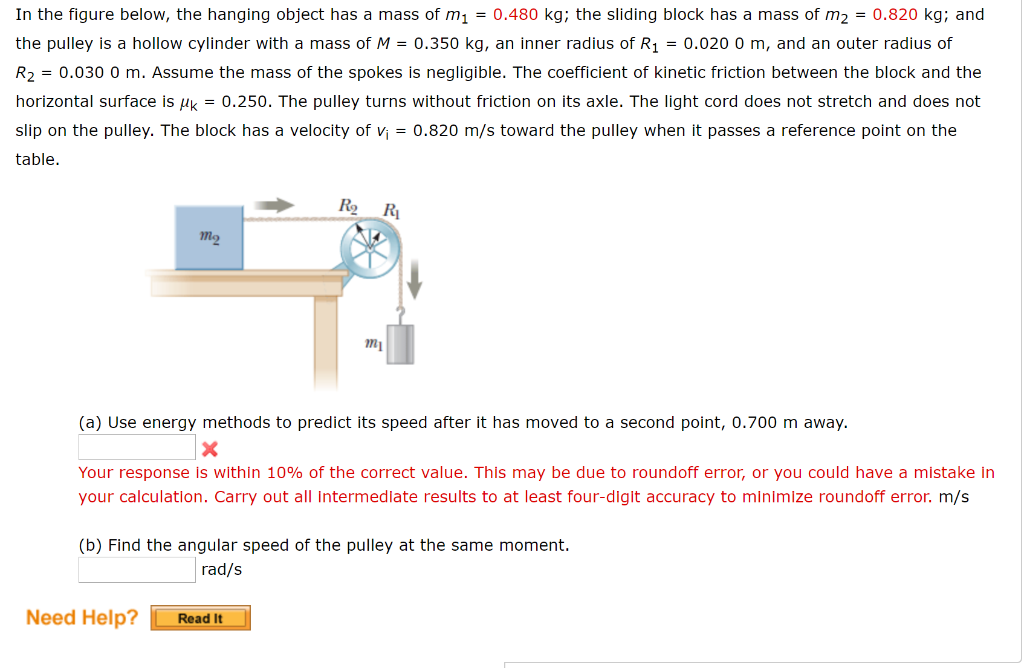In the figure below, the hanging object has a mass of m1 -0.480 kg; the sliding block has a mass of m2 0.820 kg; and the pulley is a hollow cylinder with a mass of M0.350 kg, an inner radius of R10.020 0 m, and an outer radius of R2 = 0.030 0 m. Assume the mass of the spokes is negligible. The coefficient of kinetic friction between the block and the horizontal surface is Hk0.250. The pulley turns without friction on its axle. The light cord does not stretch and does not slip on the pulley. The block has a velocity of0.820 m/s toward the pulley when it passes a reference point on the table. R2 RI mg my (a) Use energy methods to predict its speed after it has moved to a second point, 0.700 m away. Your response is within 10% of the correct value. This may be due to roundoff error, or you could have a mistake in your calculation. Carry out all Intermedlate results to at least four-digit accuracy to minlmize roundoff error. m/s (b) Find the angular speed of the pulley at the same moment. rad/s Need Help?Read It

given

m1 = 0.48 kg

m2 = 0.82 kg

M = 0.35 kg

R1 = 0.02 m

R2 = 0.3 m

k = 0.25

vi = 0.82 m/sec

a )

d = 0.7 m away

I = 0.5 M ( R12 + R22 )

= 0.5 x 0.35 x ( 0.022 + 0.032 )

= 0.0002275

K1i = 1/2 m1vi2

= 0.5 x 0.48 x 0.822

= 0.1613 J

U1i = m1 g d

= 0.48 x 9.8 x 0.7

U1i = 3.2928 J

K2i = 1/2 m2 vi2

= 0.5 x 0.82 x 0.822

= 0.275 J

Kroti = 1/2 I wi2

= 0.5 x 0.0002275 x ( vi/R2 )2

= 0.5 x 0.0002275 x ( 0.82/0.03 )2

Kroti = 0.0849 J

the frictional force fk = k m2 g

= 0.25 x 0.82 x 9.8

fk = 2 N

applying law of conservation energy is

( K1i + K2i + U1i + U2i + Krot i ) - fk d = K1f + K2f + Krot f

( 0.1613 + 0.275 + 3.2928 + 0 ) - 2 x 0.7 = 0.5 x 0.48 x vf2 + 0.5 x 0.82 x vf2 + 0.5 x

0.0002275 x ( vf / R2 )2

2.3291 = 0.24 x vf2 + 0.41 x vf2 + 0.126 vf2

0.24 x vf2 + 0.41 x vf2 + 0.126 vf2 = 2.3921

vf2 = 2.3921/0.776

vf2 = 3.0826

vf = 1.755 m/sec

b )

wf = vf / R2

= 1.755 / 0.03

#### Earn Coin

Coins can be redeemed for fabulous gifts.

Similar Homework Help Questions
• ### In the figure below, the hanging object has a mass of m1 -0.480 kg; the sliding block has a mass ...In the figure below, the hanging object has a mass of m1 -0.480 kg; the sliding block has a mass of m2 0.820 kg; and the pulley is a hollow cylinder with a mass of M0.350 kg, an inner radius of R10.020 0 m, and an outer radius of R2 = 0.030 0 m. Assume the mass of the spokes is negligible. The coefficient of kinetic friction between the block and the horizontal surface is Hk0.250. The pulley turns without...

• ### In the figure below, the hanging object has a mass of m, = 0.480 kg; the...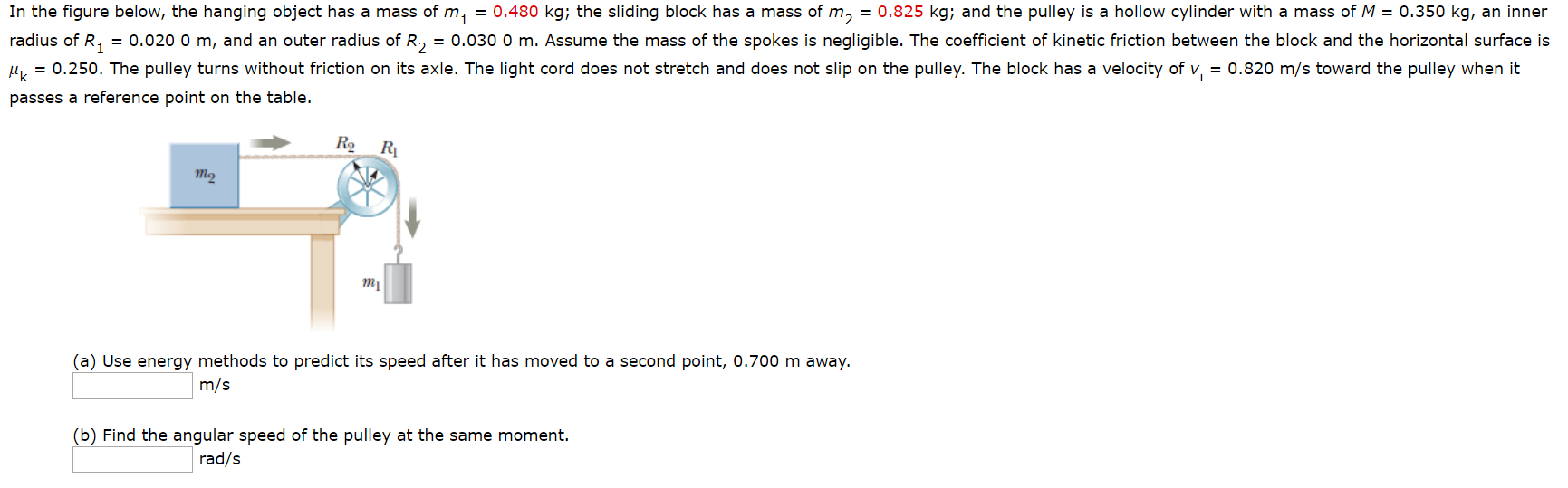In the figure below, the hanging object has a mass of m, = 0.480 kg; the sliding block has a mass of m, = 0.825 kg; and the pulley is a hollow cylinder with a mass of M = 0.350 kg, an inner radius of R4 = 0.020 0 m, and an outer radius of R, = 0.030 0 m. Assume the mass of the spokes is negligible. The coefficient of kinetic friction between the block and the horizontal surface...

• ### In the figure below, the hanging object has a mass of m_1 = 0.400 kg; the...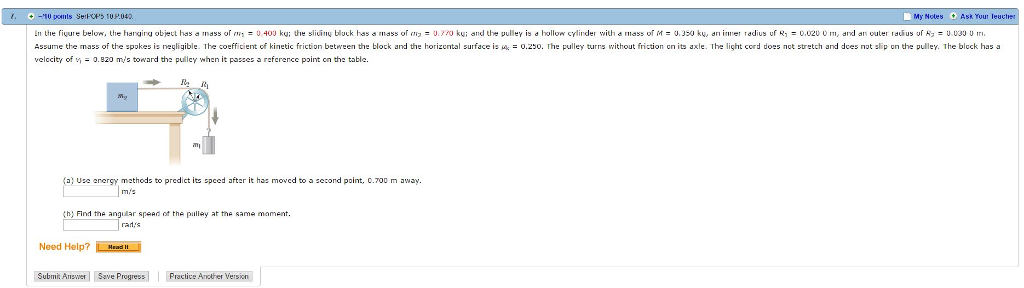In the figure below, the hanging object has a mass of m_1 = 0.400 kg; the sliding block has a mass of m_2 = 0.770 kg; and the pulley is a hollow cylinder with a mass of M = 0.350 kg, an inner radius of R_1 = 0.020 0 m, and an cuter radius of R_3 = 0.030 0 m. Assume the mass of the spokes is negligible. The coefficient of kinetic friction between the block and the horizontal surface...

• ### A hanging weight, with a mass of m1 = 0.365 kg, is attached by a rope...

A hanging weight, with a mass of m1 = 0.365 kg, is attached by a rope to a block with mass m2 = 0.825 kg as shown in the figure below. The rope goes over a pulley with a mass of M = 0.350 kg. The pulley can be modeled as a hollow cylinder with an inner radius of R1 = 0.0200 m, and an outer radius of R2 = 0.0300 m; the mass of the spokes is negligible. As...

• ### A hanging weight, with a mass of me = 0.350 kg, is attached by a rope...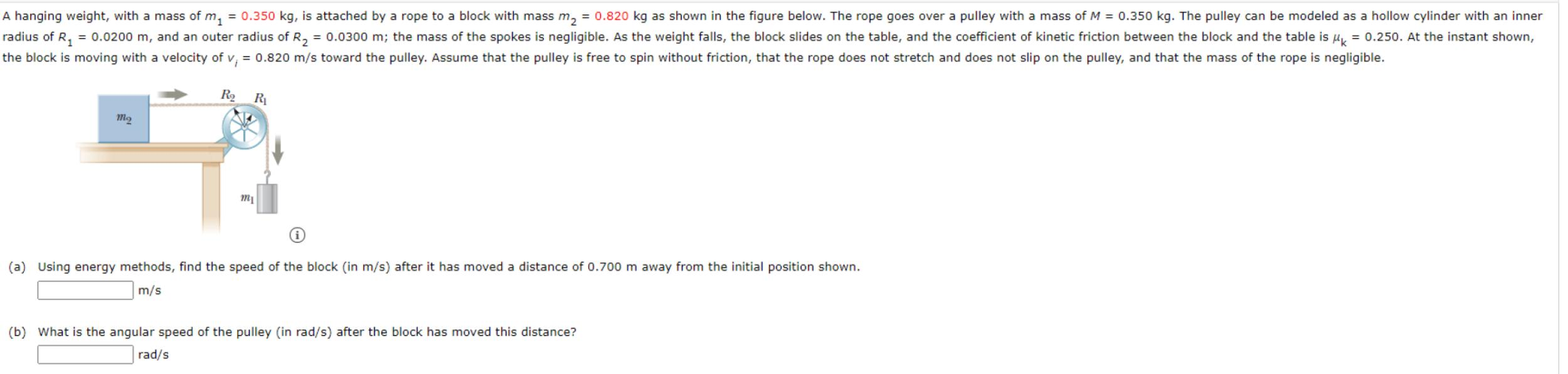A hanging weight, with a mass of me = 0.350 kg, is attached by a rope to a block with mass m2 = 0.820 kg as shown in the figure below. The rope goes over a pulley with a mass of M = 0.350 kg. The pulley can be modeled as a hollow cylinder with an inner radius of R2 = 0.0200 m, and an outer radius of R2 = 0.0300 m; the mass of the spokes is negligible. As...

• ### Given the system of 2 masses attached by a rope over a hollow pulley, predict the speed after...In the figurebelow, the hanging object has a mass of m1 = 0.500 kg; the sliding block has a mass of m2 = 0.770 kg; and the pulley is a hollow cylinder with a mass of M = 0.350kg, an inner radius of R1 = 0.020 0 m, and an outer radius of R2 = 0.030 0 m. Assume the mass of the spokes is negligible. The coefficient of kinetic frictionbetween the block and the horizontal surface is μk =...

• ### pulleys with mass, planes with friction, physics whiz dream problem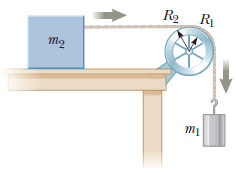In the figure below, the hanging object has a mass of m1 = 0.385 kg; the sliding block has a mass of m2 = 0.780 kg; and the pulley is a hollow cylinder with a mass ofM = 0.350 kg, an inner radius of R1 = 0.020 0 m, and an outer radius of R2 = 0.030 0 m. Assume the mass of the spokes is negligible. The coefficient of kineticfriction between the block and the horizontal surface is µk...

• ### Why is my cacluation off?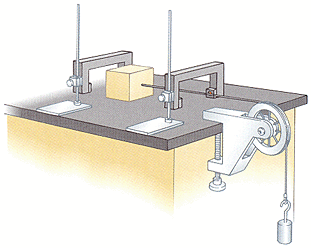The sliding block has a mass of 0.770 kg,the counterweight has a mass of 0.430 kg,and the pulley is a hollow cylinder with a mass of 0.350 kg, aninner radius of 0.020m, and an outer radius of 0.030 m. Thecoefficient of kinetic friction between the block and thehorizontal surface is 0.250. The pulley turns without friction onitsaxle. The light cord does not stretch and does not slip on thepulley. The block has a velocity of 0.820 m/s toward the pulleywhen...

• ### A hanging weight, with a mass of m, -0.370, is attached by a rope to a...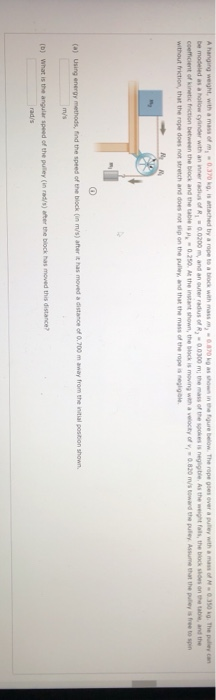A hanging weight, with a mass of m, -0.370, is attached by a rope to a block with mass-0070 Ahow in the gure below. The rope goes over a pulley with a f.350 G. The pulley can be modeled as a hollow cylinder with an inner radius of R = 0.0200, and an outer radius of R, -0.0300 m the mass of the spoke is negligible. As the weight fals, the black shoes on the table, and the coefficient of...

• ### Hanging Mass

A block off mass m resting on a 20 deg slope. The block has coefficients of friction static = .8 and kinetic = .5 with the surface. It is connected via a masslessstring over a massless, frictionless pulley to a hanging block of mass 2.0 kg.a) What is the minimum mass m that will stick and not slipb) If this minimum mass is nudged ever so slightly, it will start being pulled up the incline.What acceleration will it have.

Free Homework App